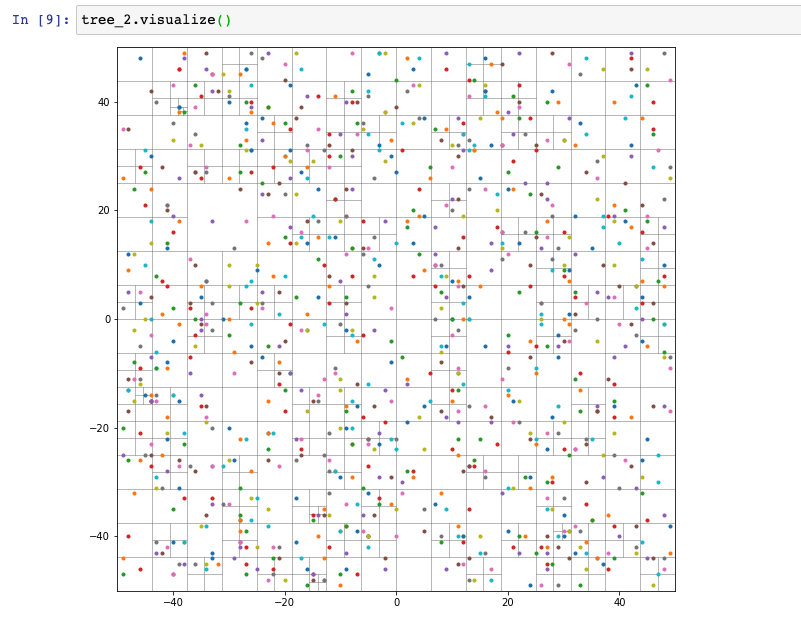Quadtrees are a useful data structure for sparse datasets where the location/position of the data is important. They’re especially good for spatial indexing & image processing.## Usage¶

```>>> import quads
...     (0, 0),  # The center point
...     10,  # The width
...     10,  # The height
... )

# You can choose to simply represent points that exist.
>>> tree.insert((1, 2))
True
# ...or include extra data at those points.
True

# You can search for a given point. It returns the point if found...
>>> tree.find((1, 2))
Point(1, 2)

# Or `None` if there's no match.
>>> tree.find((4, -4))
None

# You can also find all the points within a given region.
>>> bb = quads.BoundingBox(min_x=-1, min_y=-2, max_x=2, max_y=2)
>>> tree.within_bb(bb)
[Point(1, 2)]

# You can also search to find the nearest neighbors of a point, even
# if that point doesn't have data within the quadtree.
>>> tree.nearest_neighbors((0, 1), count=2)
[
Point(1, 2),
Point(4, -4),
]

# And if you have `matplotlib` installed (not required!), you can visualize
# the tree.
```

## Installation¶

```\$ pip install quads
```

## Requirements¶

• Python 3.7+ (untested on older versions but may work)